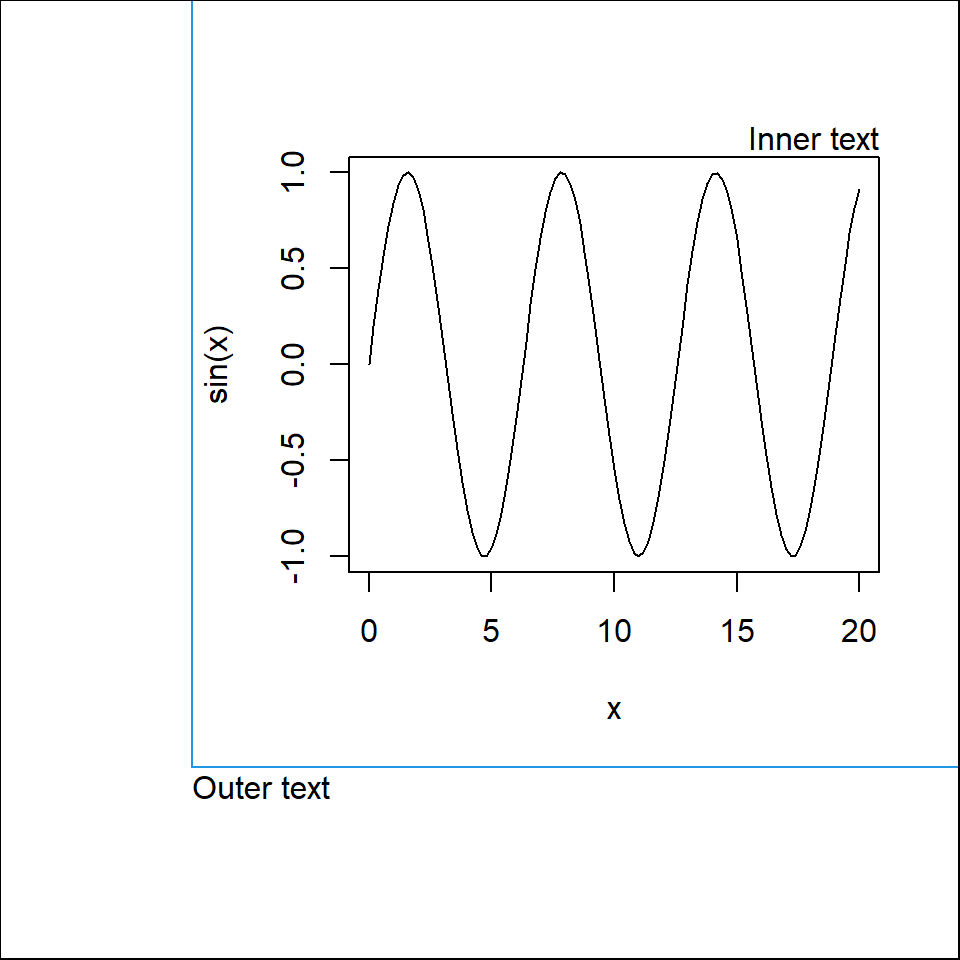# Margins in R

## Inner margins: `mar` and `mai`

The margins of a plot can be customized with the `mar` graphical parameter, if the margin is measured in margin lines and `mai` if the margin is measured in inches.

These graphical parameters are vectors of the form `c(bottom, left, top, right)`, where each element represent the margin of each side of the plot (in margin lines or in inches). Note that you can access the current values with `par("mar")` or `par("mai")`.

The order of the elements of `mar` and `mai` vectors are `bottom`, `left`, `top` and `right`. A good way to remember is remembering the word `b`o`l`s`t`e`r`.

mar (margin lines)

By default `par(mar = c(5, 4, 4, 2) + 0.1)`.

In the figure you can see how many lines fit on each side of the plot. You can increase or decrease the number of lines for each side. Note that the 0.1 is just for adding an extra space to fit everything inside the plot area.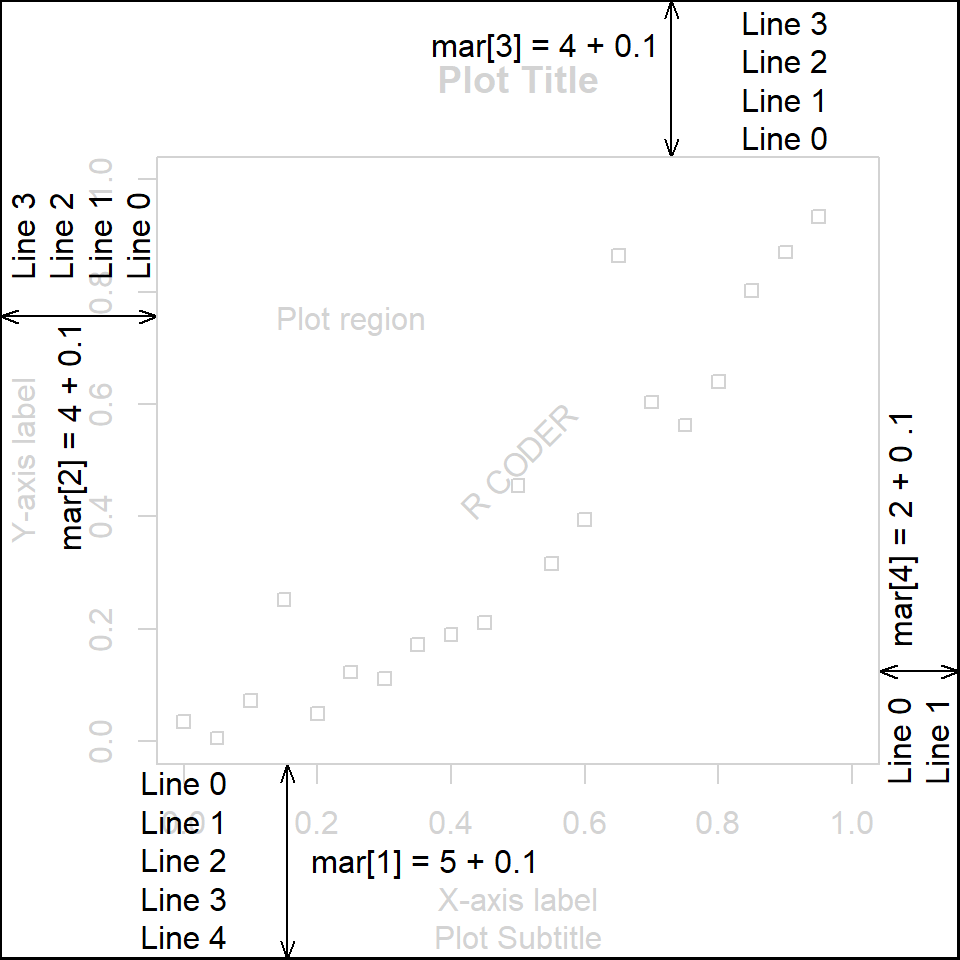mai (inches)

By default `par(mar = c(1, 0.8, 0.8, 0.4) + 0.02)`

If you prefer set the margins in inches instead of in margin lines modify this graphical parameter.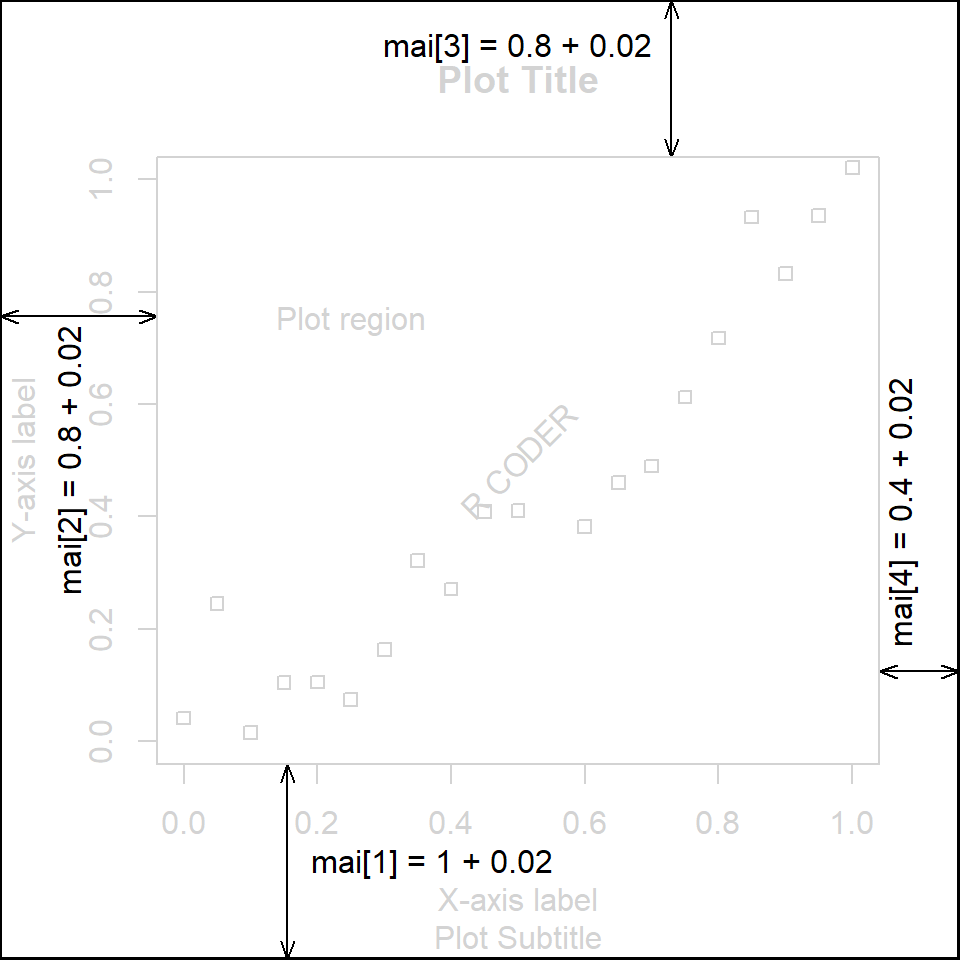### Example 1. Using `mar`

In this example we are going to remove the top and right margins. Note that we added a box to the figure so you can see the difference.

Default margins

``````# Default
curve(sqrt, 0, 20)
box("figure", col = 4)``````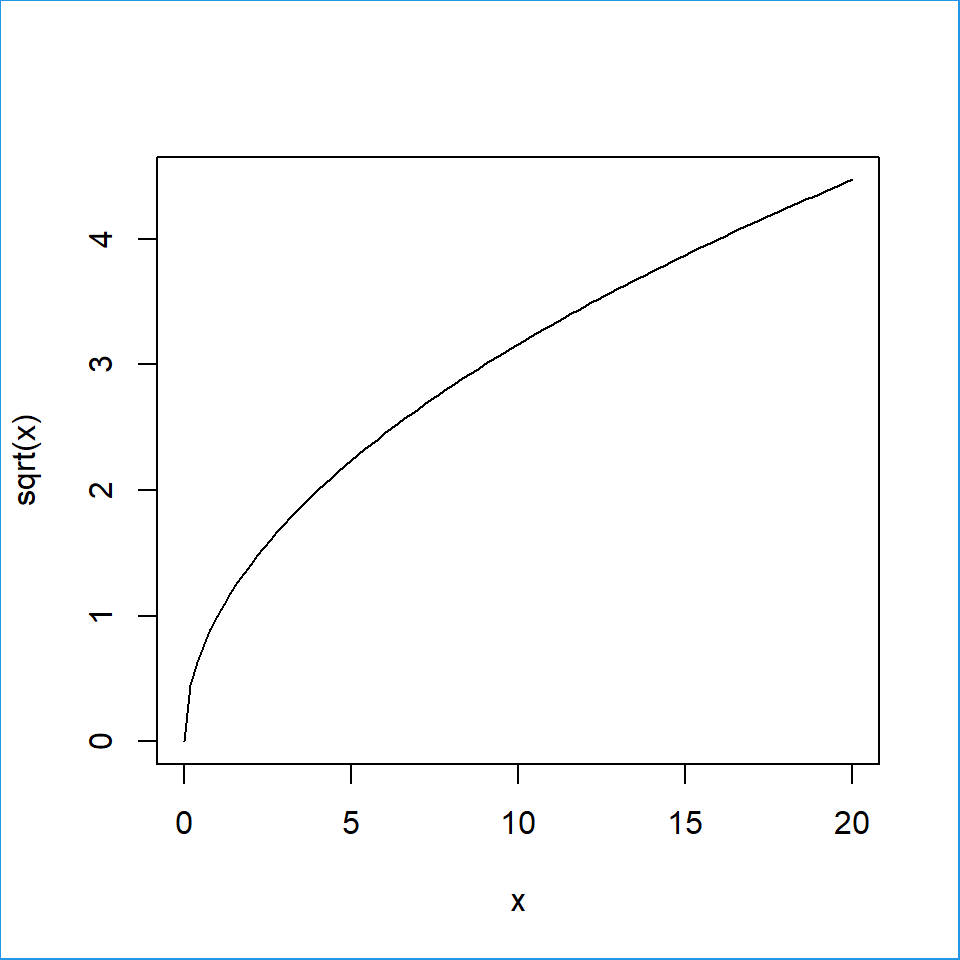Remove top and right margins

``````par(mar = c(par("mar"), par("mar"), 0, 0))
curve(sqrt, 0, 20)
box("figure", col = 4)``````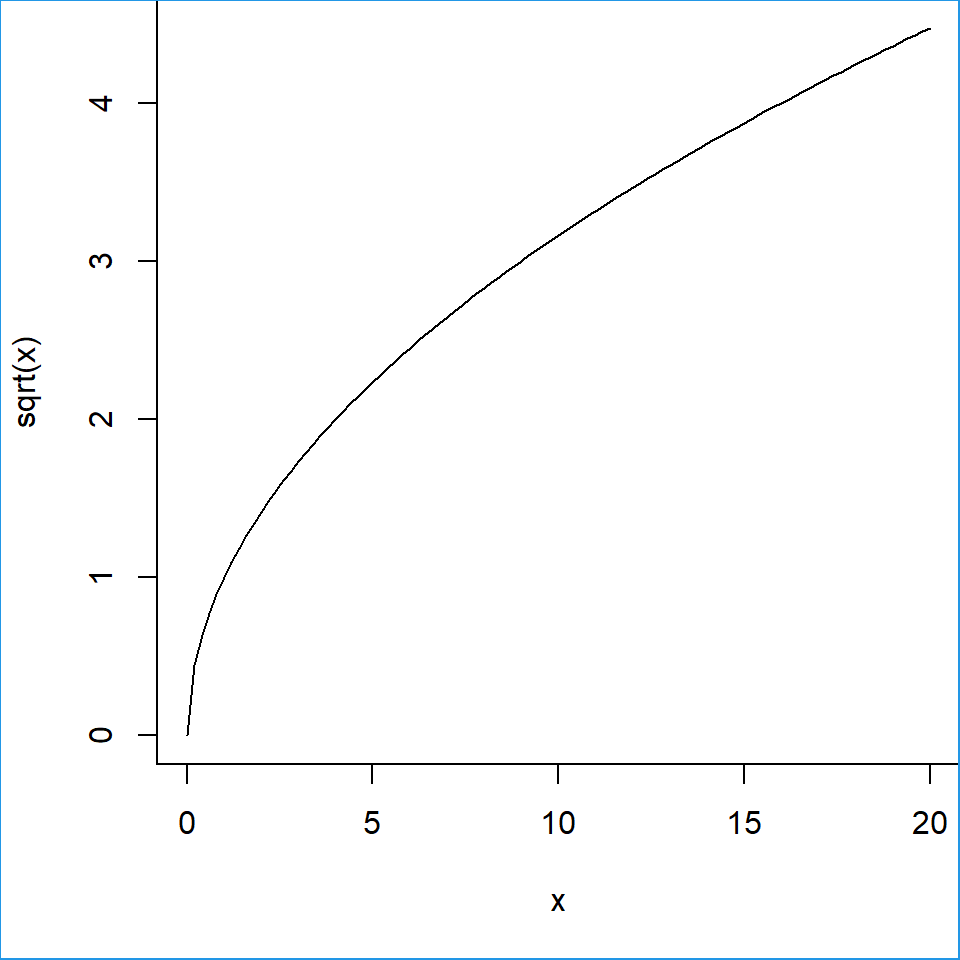### Example 2. Using `mai`

In this example we are increasing all the margins. Once again, we added a box to the figure so you can see the difference.

Default margins

``````# Default
curve(sqrt, 0, 20)
box("figure", col = 4)``````Longer margins

``````par(mai = c(1.5, 1.5, 1.5, 1.5))
curve(sqrt, 0, 20)
box("figure", col = 4)``````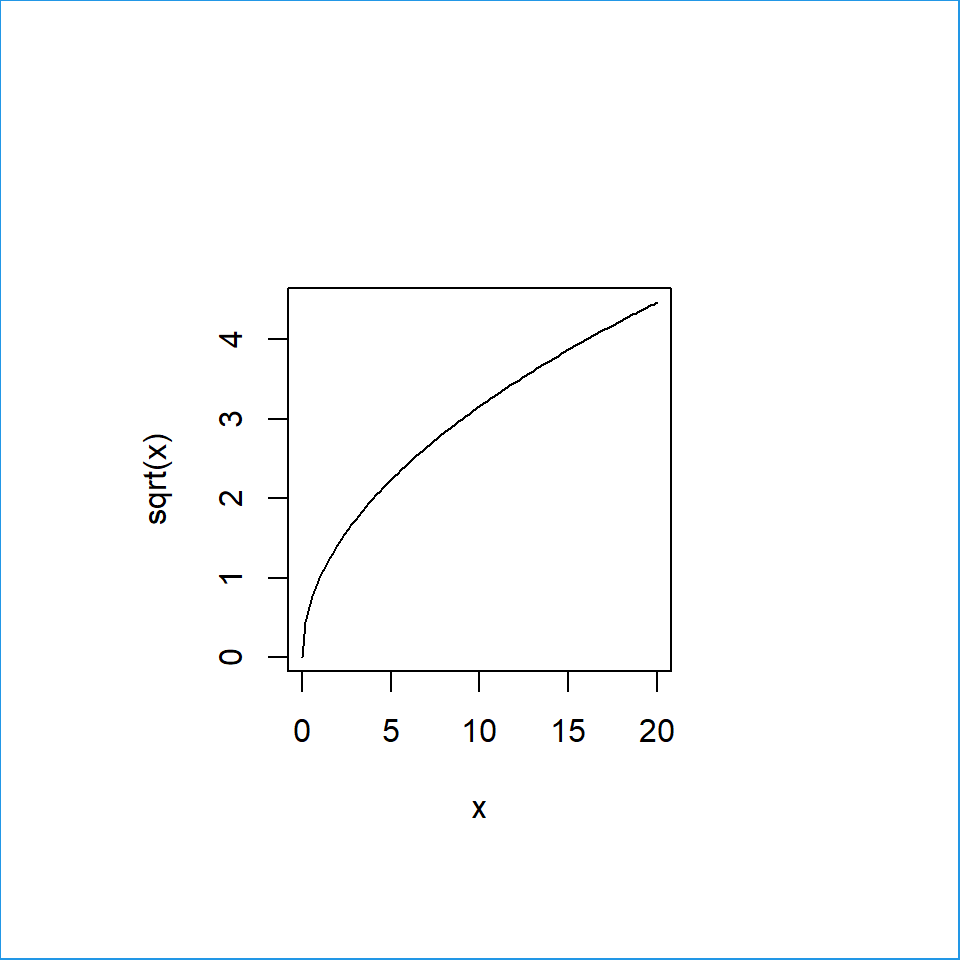## Outer margins: `oma` and `omi`

Outer margins can be specified in the same way as `mar` and `mai`. You can set the margin in margin lines with `oma` or in inches with `omi`. The outer margins are specially useful for adding text to a combination of plots (a single title for multiple plots).

You can access the current outer margins with `par("oma")` and `par("omi")`.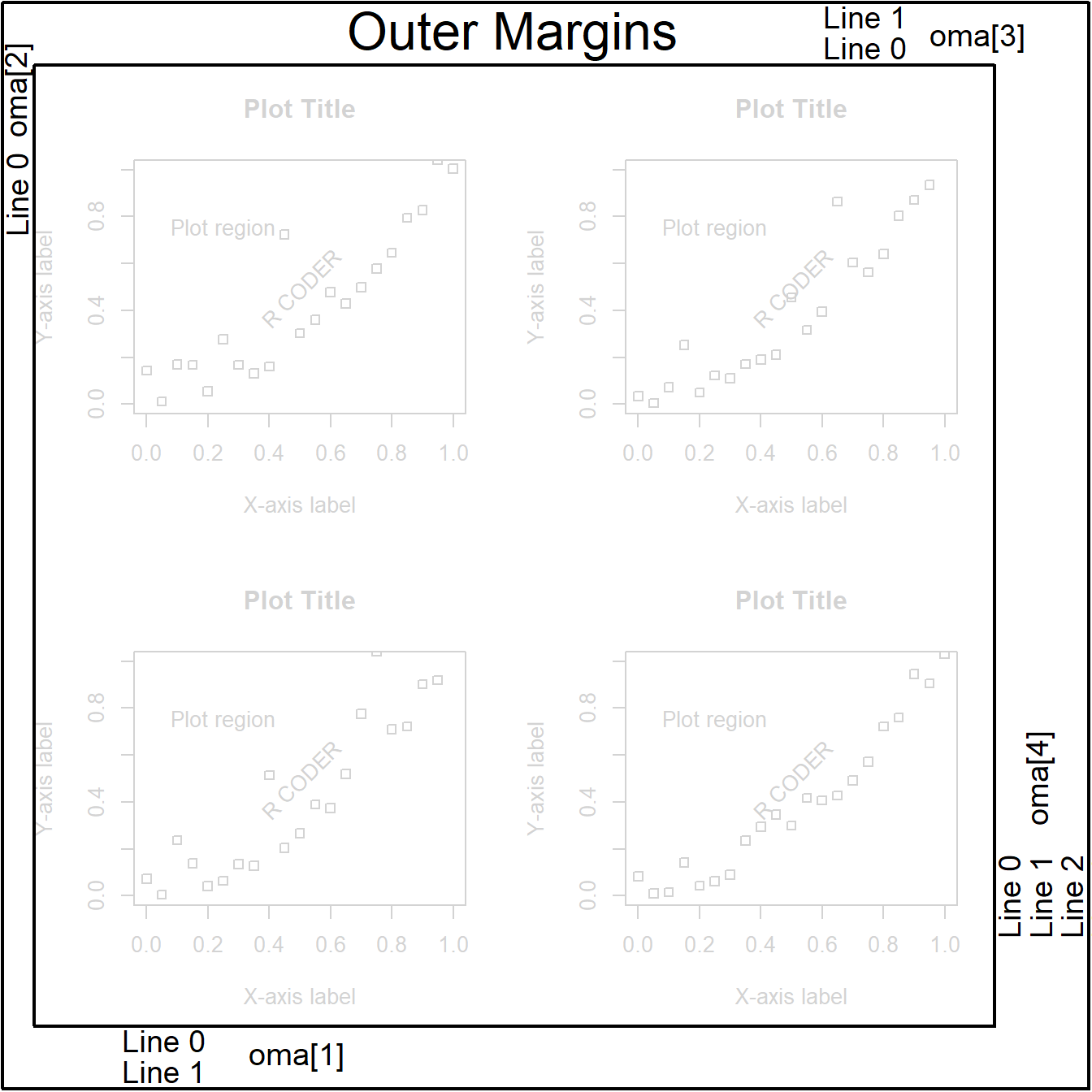By default, `par(oma = c(0, 0, 0, 0))`. In this example we set `par(oma = c(2, 1, 2, 3) + 0.1)`, so two lines are displayed below the plot, one on the left, two on the top and three on the right.

### Example 1. Using `oma`

In the following example we added four margin lines on each side, so four stacked lines of text will fit out of the figure. The default figure boundaries are represented with a blue box and the new ones with a black box.

Default outer margins

``````# Default
curve(sin, 0, 20)

# Figure box
box("figure", col = 4)
# Text
mtext("Inner text",
side = 3, adj = 1)``````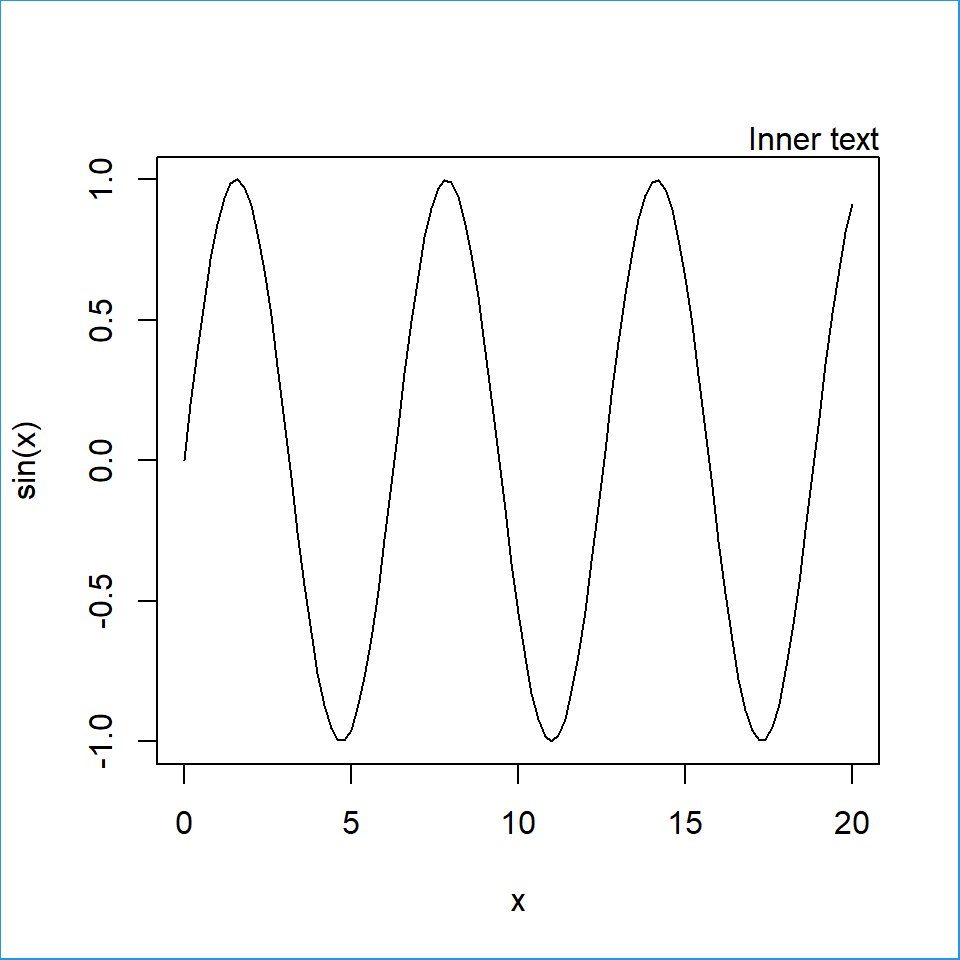Longer outer margins

``````par(oma = c(4, 4, 4, 4)) # Outer margins
curve(sin, 0, 20)        # Plot
box("figure", col = 4)   # Box figure
mtext("Inner text", side = 3, adj = 1)
mtext("Outer text", side = 3, adj = 1,
outer = TRUE)
par(oma = c(0, 0, 0, 0)) # Restore margins
box("figure", col = 1)   # Outer box``````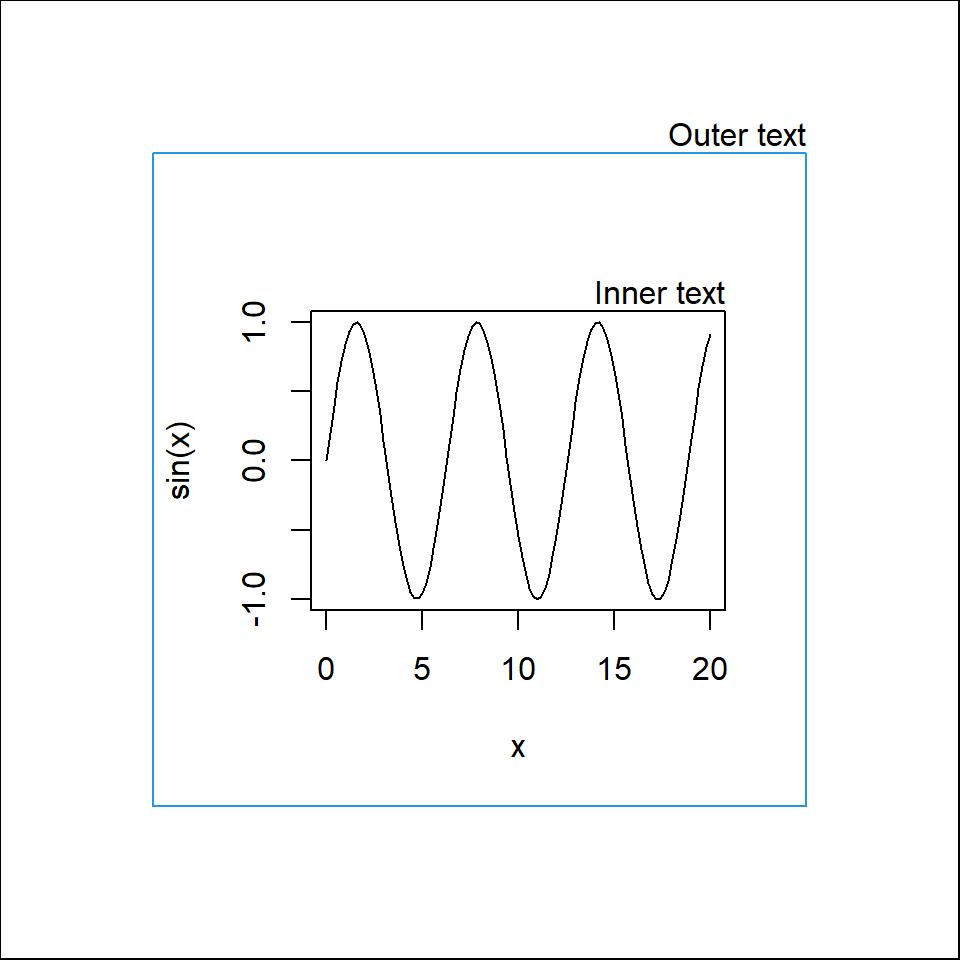### Example 2. Using `omi`

In this example we added 1 inch on the bottom and left margins. Once again, the boundaries of the default figure are represented with a blue box and the new ones with a black box, so you can visualize the difference.

Default outer margins

``````# Default
curve(sin, 0, 20)

# Figure box
box("figure", col = 4)

# Text
mtext("Inner text", side = 3, adj = 1)``````Bottom and left outer margins

``````par(omi = c(1, 1, 0, 0)) # Outer margins
curve(sin, 0, 20)        # Plot
box("figure", col = 4)   # Inner box
mtext("Inner text", side = 3, adj = 1)
mtext("Outer text", side = 1, adj = 0,
outer = TRUE)
par(oma = c(0, 0, 0, 0)) # Restore margins
box("figure", col = 1)   # Outer box``````